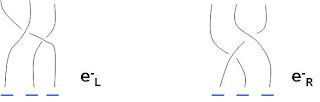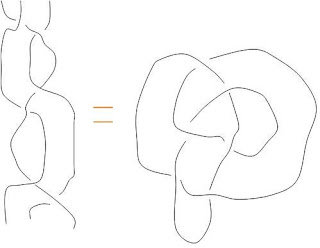## Riemann Revisited II

I’m so excited by this claim of Tribikram Pati! I haven’t read the paper yet, but quoting from the abstract: our analysis shows that the assumption of the truth of the Riemann Hypothesis leads to a contradiction. Maybe he’s right! After all, we’ve seen that the zeta function really shouldn’t be studied within the confines of Boolean logic. But then there is already a post by Julia Kuznetsova claiming to have found the (almost inevitably present) flaw. A reply by a K. L. Lange to this criticism, supporting the proof, states, “So the main idea of [Pati] was to show, that we need that delta to prove RH, but there is no delta, so we cannot prove RH after all …” In other words, the paper may well show that there is no proof of the Hypothesis within standard analysis. That doesn’t sound surprising at all.

If we built an L function on the surreals in the M Theory operadic landscape, would we care about the ordinary Riemann zeta function? Yes, of course we would, because it’s very interesting! And the M Theory L function would contain the Riemann one anyway. I have reluctantly come to the conclusion that a decent definition for n-logoses really must sort out the meaning of Analysis in category theory. This can’t be done the 1-topos way. At least surreals have infinitesimals. And now I should probably confess that I always had great difficulties with analysis. Well, I also have great difficulties with Geometry, Algebra and Logic, which is one good reason for studying category theory, because it rolls them all into one! But as Donaldson, Perelman and many other mathematicians have shown, beautiful proofs these days need analysis.

## Riemann Revisited

On Lieven Le Bruyn’s recommendation, I decided to read du Sautoy’s book The Music of the Primes. It is exceedingly enjoyable and informative (except for the physics glitches).

The anecdotes range from Gauss’s discovery of Ceres to Dyson’s fortuitous meeting with Montgomery. I particularly liked the suspenseful introduction based on Bombieri’s famous April Fool’s Joke of 1997, stating that the Riemann hypothesis had been proved by a young physicist. He wrote, “the physics corresponding to a near absolute zero ensemble of a mixture of anyons and morons with opposite spins.” Perhaps we should really name a particle the moron in honour of Bombieri’s great humour!

Update: Wow! Lieven has pointed me to this post about a possible disproof of the Riemann hypothesis. Or rather, a proof that it cannot be proved within set theory? That would be just AMAZING!!! Here is the paper in question. Must go check it out…

## Construction Site

What is an n-category? Something indexed by an ordinal n? But if we want to talk about higher toposes we can’t begin by assuming that we know what an ordinal is! The 1-topos Set conveniently has an object of ordinals, characterised by a successor function. But n-logoses, being fundamental entities, cannot be based on diagrams in Set.

Another place where ordinals are found is in simplicial sets, which are Set valued functors from a category Ord of finite ordinals and order preserving functions. Think of real geometric n-simplices on n vertices, like the tetrahedron for the ordinal 3. But once again, in defining Ord, we assumed that we knew what an ordinal was. The only way out of this dilemma seems to be to construct ordinals as we define n-logoses. For an elementary topos, for example, we certainly need the concepts 0 and 1 (empty set and one point set), but the concept of the ordinal 2 could wait until we need it for the triangle of ternary logic. This suggests using this triangle whenever we might be tempted to use the ordinal 2. For example, a simplicial category Ord can’t be defined until we have a notion of $\omega$-logos. Sounds like an awful lot of work!

The ancients knew that for a set theoretic cardinal n, there was always a decomposition into prime factors. This is the fundamental theorem of arithmetic, but it does not hold for general fields. Anyway, there’s a problem with Euclid’s original proof by contradiction: it’s not constructive. Just because a statement is false, doesn’t mean we can assume that its converse is true! And what is a converse? We’ve seen that 3-logoses require a notion of ternary complementation. Somewhere in the land of $\omega$-logoses there is an awfully complicated notion of primeness.

If any of this makes sense, it suggests that the infinite branchings of the surreal tree follow the process of logosification. Looking at the positive half only, we see a simple Y tree at the level of 2. At the branches, the number 2 has been defined along with the number 1/2. We conclude that the concept of 2-category must be accompanied by a T-dual concept of 1/2-category!

Maybe the appearance of associahedra as coherence laws for n-categories would be a little less surprising in this setup. That is, an (n+2)-leaved 1-tree polytope appears in the definition of n-category, as n goes to infinity.

## M Theory Lesson 59

One of the most information packed 600+ page tomes in the library here is Sphere Packings, Lattices and Groups by J. H. Conway and N. J. A. Sloane. It looks at the 24 dimensional Leech lattice in numerous ways.

Chapter 1 covers the basics of lattice theory and sphere packings. On page 5 it is noted that for a hexagonal plane tiling, rather than basis vectors $(1,0)$ and $(0.5, 0.5 \sqrt{3})$ in two dimensions, it is useful to use the simple three dimensional coordinates $(1,-1,0)$ and $(0,1,-1)$, which lie on the plane $x + y + z = 0$. This basis gives a Dynkin diagram labelling of the $A_2$ root lattice. It corresponds to the densest sphere packing in two dimensions with a density of $\frac{\pi}{\sqrt{12}}$.

The theta function of an integral lattice is related to modular forms. For example, for the Leech lattice the series takes the form

$1 + 196560 q^4 + 16773120 q^6 + \cdots$

which follows from a term for the $E_8$ theta series minus another term which is 720 times the Ramanujan series

$q^2 – 24 q^4 + 252 q^6 -1472 q^8 + \cdots$

Defining $\theta (\tau) = \sum_{- \infty}^{\infty} e^{\pi i \tau n^{2}}$, one can use Riemann’s observation that

$\theta (\frac{-1}{\tau}) = \sqrt{- i \tau} \theta (\tau)$

to prove the functional equation for the zeta function. Recall that in M theory this property of the zeta function is intimately related to the physical duality of Space and Time. In a logos style constructive approach to zeta functions it is therefore natural to view lattices and theta series as useful tools for the geometrization of operad polytopes.

Note that the currently popular j invariant may be expressed easily in terms of theta series as

$j (\tau) = 32 \frac{(\theta (\tau)^8 + \theta_{01} (\tau)^8 + \theta_{10} (\tau)^8)^{3}}{(\theta (\tau) \theta_{01} (\tau) \theta_{10} (\tau))^{8}}$

which comes from basic elliptic function theory, but smells of triality, I think.

## Blog Notice

What everybody was waiting for … Borcherd’s new blog! And the first post is on the uselessness of Planck units. He’s already on my blogroll.

## M Theory Lesson 58A 5-edged 2-level tree can have at most 4 input leaves. These trees define two dimensional polytopes as shown. The pentagon is familiar from 1-operad tilings, or as the Mac Lane pentagon, and the hexagon from the braiding rule for braided monoidal categories. Only polygons of six sides or less are capable of tiling the plane regularly. Here are some purely pentagonal tilings.

Higher dimensional analogues of the hexagon polytope are known as permutohedra, because they describe permutations on $(d + 1)$ letters. Loday showed that by cutting hypercubes with hyperplanes one can naturally obtain both associahedra and then permutohedra in any dimension. Postnikov et al have done a lot of work on the combinatorics of generalised permutohedra.

As a shameless thief of pretty pictures, today I offer the reader the $6_3$ knot on the Klein surface! A six punctured Klein surface has a moduli space of real dimension 24, which is a number we like a lot.## M Theory Lesson 57

Recall that the Bilson-Thompson diagrams for left and right handed electrons are formed with three strands, each with a full negative twist representing a one third charge.Ignoring the twists, the simplest braid composition of the form $e_L e_R e_L$ looks like this:This knot is called a $6_3$ knot, according to the amazing online knot atlas, which lists the polynomial invariants for this knot. For example, the Jones polynomial takes the form

$-q^3 + 2 q^2 -2q + 3 -2 q^{-1} + 2 q^{-2} – q^{-3}$

Since all negative twists add up on going around the knot, there is a total ninefold twist. There is now a button to the knot atlas on the sidebar.

## The Old Man

Given anonymous’ interesting suggestion to use honeycombs to study the rings of Saturn, I couldn’t resist posting this false colour NASA/JPL/SSI Cassini image, stolen from Universe Today.This view is from above the hot North pole (in shadow) where we recently saw a hexagon structure.

## M Theory Lesson 56

Returning to Heisenberg’s 1925 paper, we find the basic honeycomb sum rule (on page 3) as the quantum mechanical version of the classical relation

$\omega (n,a) + \omega (n,b) = \omega (n,a+b)$

To a category theorist this classical relation looks like a triangle, where two sides compose to give the third. Moreover, since $n$ is fixed, we may view $\omega(n,-)$ as a functor from a category containing the triangle $(a,b,a+b)$. This source category has addition as composition. There is only one object. In fact, it is an Abelian group in the form of a category on one object. The target category, containing the frequencies as arrows, is also an Abelian group, so the functor $\omega (n,-)$ is just a group homomorphism.

Following Heisenberg, the quantum relation takes the form

$\omega (n, n-a) + \omega (n-a, n-a-b) = \omega (n, n-a-b)$

Setting $a = 0$ we see that $\omega (n,n)$ acts as a (left) identity for frequency composition, replacing the classical $\omega (n,0)$. It is now necessary to consider a variable $n$ in the source category of the classical case, so frequencies are best expressed as 2-arrows between 1-arrow integers. Thus the honeycomb diagrams in the plane are associated with two dimensional categorical structures. Alternatively, and loosely speaking, a set theoretic real number classical physics suggests a categorical complex number quantum physics.